Win up to 100% scholarship on Aakash BYJU'S JEE/NEET courses with ABNAT Win up to 100% scholarship on Aakash BYJU'S JEE/NEET courses with ABNAT

# JEE Main 2022 June 25 – Shift 2 Physics Question Paper with Solutions

To make it easier for students to learn and understand each topic covered in the question paper, our subject matter experts have created the solutions to each question with detailed explanations. Students will be able to quickly and easily solve the problems by referring to the JEE Main 2022 June 25 – Shift 2 Physics Question Paper with Solutions on this page. In essence, they will be able to effectively study for the examination. Students can also obtain the PDF of the JEE Main 2022 Question Papers for June 25 – Shift 2 Physics from this page.

## JEE Main 2022 Physics Question Paper and Solutions – June 25th Shift 2

#### SECTION – A

Multiple Choice Questions: This section contains 20 multiple choice questions. Each question has 4 choices (1), (2), (3) and (4), out of which ONLY ONE is correct.

1. Given below are two statements. One is labelled as Assertion A and the other is labelled as Reason R.

Assertion A: Two identical balls A and B thrown with same velocity ’u’ at two different angles with horizontal attained the same range R. If A and B reached the maximum height h1 and h2 respectively, then

$$\begin{array}{l}R=4\sqrt{h_1h_2}.\end{array}$$

Reason R: Product of said heights.

$$\begin{array}{l}h_1h_2=\left ( \frac{u^2sin^2\theta}{2g} \right ).\left ( \frac{u^2cos^2\theta}{2g} \right )\end{array}$$

(A) Both A and R are true and R is the correct explanation of A.

(B) Both A and R are true but R is NOT the correct explanation of A.

(C) A is true but R is false.

(D) A is false but R is true.

Sol.

$$\begin{array}{l}h_1=\frac{u^2sin^2\theta}{2g}\end{array}$$
$$\begin{array}{l}h_2=\frac{u^2cos^2\theta}{2g}\end{array}$$
$$\begin{array}{l}\therefore \sqrt{h_1h_2}=\frac{u^2sin\theta \cos\theta}{2g}\end{array}$$
$$\begin{array}{l}=\frac{R}{4}\end{array}$$
$$\begin{array}{l}\Rightarrow R=4\sqrt{h_1h_2}\end{array}$$

2. Two buses P and Q start from a point at the same time and move in a straight line and their positions are represented by XP(t) = αt + βt2 and XQ(t) = ftt2. At what time, both the buses have same velocity?

$$\begin{array}{l}(A)\ \frac{\alpha -f}{1+\beta}\end{array}$$
$$\begin{array}{l}(B)\ \frac{\alpha +f}{2(\beta-1)}\end{array}$$
$$\begin{array}{l}(C)\ \frac{\alpha +f}{2(1+\beta)}\end{array}$$
$$\begin{array}{l}(D)\ \frac{f-\alpha}{2(1+\beta)}\end{array}$$

Sol. XP = αt + βt2

XQ = ftt2

VP = α + 2βt

VQ = f – 2t

VP = VQ

⇒ α + 2βt = f – 2t

$$\begin{array}{l}\Rightarrow t=\frac{f-\alpha}{2(1+\beta)}\end{array}$$

3. A disc with a flat small bottom beaker placed on it at a distance R from its center is revolving about an axis passing through the center and perpendicular to its plane with an angular velocity ω. The coefficient of static friction between the bottom of the beaker and the surface of the disc is μ. The beaker will revolve with the disc if :

$$\begin{array}{l}(A)\ R\leq \frac{\mu g }{2\omega ^2}\end{array}$$
$$\begin{array}{l}(B)\ R\leq \frac{\mu g }{\omega ^2}\end{array}$$
$$\begin{array}{l}(C)\ R\geq \frac{\mu g }{2\omega ^2}\end{array}$$
$$\begin{array}{l}(D)\ R\geq \frac{\mu g }{\omega ^2}\end{array}$$

Sol. To move together

ω2R ≤ μg

$$\begin{array}{l}\Rightarrow R\leq \frac{\mu g}{\omega ^2}\end{array}$$

4. A solid metallic cube having total surface area 24 m2 is uniformly heated. If its temperature is increased by 10°C, calculate the increase in volume of the cube.

(Given α = 5.0 × 10–4 °C–1).

(A) 2.4 × 106 cm3

(B) 1.2 × 105 cm3

(C) 6.0 × 104 cm3

(D) 4.8 × 105 cm3

Sol. 6 × l2 = 24

l = 2 m

$$\begin{array}{l}\therefore\frac{\Delta V}{V}=3\times\frac{\Delta l}{l}\end{array}$$

⇒ ΔV = 3 × (αΔT) × V

= 3 × 5 × 10–4 × 10 × (8)

= 120 × 10–3 m3

= 120 × 10–3 × 106 cm3

= 1.2 × 105 cm3

5. A copper block of mass 5.0 kg is heated to a temperature of 500°C and is placed on a large ice block. What is the maximum amount of ice that can melt?

[Specific heat of copper : 0.39 J g–1 °C–1 and latent heat of fusion of water : 335 J g–1]

(A) 1.5 kg

(B) 5.8 kg

(C) 2.9 kg

(D) 3.8 kg

Sol. mL = ΔQ = msΔT

$$\begin{array}{l}\Rightarrow m=\frac{5\times0.39\times10^3\times500}{335}\end{array}$$

= 2.9 kg

6. The ratio of specific heats

$$\begin{array}{l}\left ( \frac{C_p}{C_v} \right )\end{array}$$
in terms of degree of freedom (f) is given by:

$$\begin{array}{l}(A)\ \left (1+ \frac{f}{3} \right )\end{array}$$
$$\begin{array}{l}(B)\ \left (1+ \frac{2}{f} \right )\end{array}$$
$$\begin{array}{l}(C)\ \left (1+ \frac{f}{2} \right )\end{array}$$
$$\begin{array}{l}(D)\ \left (1+ \frac{1}{f} \right )\end{array}$$

Sol.

$$\begin{array}{l}\frac{C_P}{C_V}=\gamma \end{array}$$
$$\begin{array}{l}C_V=\left ( \frac{f}{2} \right )R\end{array}$$
and
$$\begin{array}{l}C_P- C_V=R\end{array}$$
$$\begin{array}{l}\Rightarrow \frac{C_P}{C}=\frac{1+f/2}{f/2}=1+\frac{2}{f}\end{array}$$

7. For a particle in uniform circular motion, the acceleration a at any point P(R, θ) on the circular path of radius R is (when θ is measured from the positive x-axis and v is uniform speed):

$$\begin{array}{l}(A)\ -\frac{V^2}{R}sin\theta \hat{i}+\frac{V^2}{R}cos\theta \hat{j} \end{array}$$
$$\begin{array}{l}(B)\ -\frac{V^2}{R}cos\theta \hat{i}+\frac{V^2}{R}sin\theta \hat{j} \end{array}$$
$$\begin{array}{l}(C)\ -\frac{V^2}{R}cos\theta \hat{i}-\frac{V^2}{R}sin\theta \hat{j} \end{array}$$
$$\begin{array}{l}(D)\ -\frac{V^2}{R}\hat{i}+\frac{V^2}{R}\hat{j} \end{array}$$

Sol.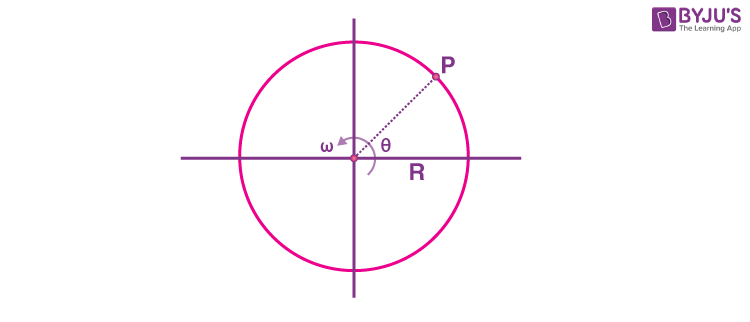As the particle in uniform circular motion experiences only centripetal acceleration of magnitude ω2R or

$$\begin{array}{l}\frac{V^2}{R}\end{array}$$
directed towards centre so from diagram.

$$\begin{array}{l}\vec{a}=\frac{V^2}{R}cos\theta(-\hat{i})+\frac{V^2}{R}sin(-\hat{j})\end{array}$$

8. Two metallic plates form a parallel plate capacitor. The distance between the plates is ‘d’. A metal sheet of thickness d/2 and of area equal to area of each plate is introduced between the plates. What will be the ratio of the new capacitance to the original capacitance of the capacitor?

(A) 2 : 1

(B) 1 : 2

(C) 1 : 4

(D) 4 : 1

Sol.

$$\begin{array}{l}C_{eq}=\frac{\epsilon _0A}{d-\frac{d}{2}+\frac{d}{2k}}=\frac{\epsilon _0A}{\frac{d}{2}}=\frac{2\epsilon _0A}{d}\end{array}$$

If

$$\begin{array}{l}C=\frac{\epsilon _0A}{d}\end{array}$$
$$\begin{array}{l}\Rightarrow C_{eq}=2C \text{ or }\ \frac{C_{new}}{C_{old}}=\frac{2}{1}\end{array}$$

9. Two cells of same emf but different internal resistances r1 and r2 are connected in series with a resistance R. The value of resistance R, for which the potential difference across second cell is zero, is:

(A) r2r1

(B) r1r2

(C) r1

(D) r2

Sol.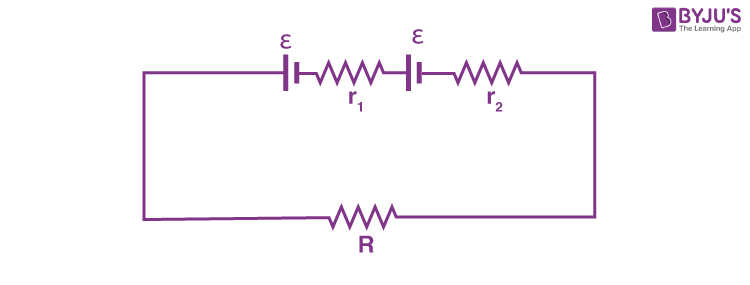$$\begin{array}{l}l=\frac{2\epsilon }{R+r_1+r_2}\end{array}$$

As per the question,

$$\begin{array}{l}\frac{2\epsilon }{R+r_1+r_2}\times r_2-\epsilon =0\end{array}$$
$$\begin{array}{l}\Rightarrow R=r_2-r_1\end{array}$$

10. Given below are two statements:

Statement-I : Susceptibilities of paramagnetic and ferromagnetic substances increase with decrease in temperature.

Statement-II : Diamagnetism is a result of orbital motions of electrons developing magnetic moments opposite to the applied magnetic field.

Choose the correct answer from the options given below:-

(A) Both Statement-I and Statement-II are true

(B) Both Statement-I and Statement-II are false

(C) Statement-I is true but Statement-II is false

(D) Statement-I is false but Statement-II is true

Sol. Statement-I is true as susceptibility of ferromagnetic and paramagnetic materials is inversely related to temperature.

Statement-II is true as because of orbital motion of electrons the diamagnetic material is able to oppose external magnetic field.

11. A long solenoid carrying a current produces a magnetic field B along its axis. If the current is doubled and the number of turns per cm is halved, the new value of magnetic field will be equal to

(A) B

(B) 2B

(C) 4B

(D) B/2

Sol. B = μ0ni

Now i → 2i

And

$$\begin{array}{l}n\to \frac{n}{2}\end{array}$$
$$\begin{array}{l}B’=\mu _0\frac{n}{2}\times2i=\mu _0ni=B\end{array}$$

12. A sinusoidal voltage V(t) = 210 sin 3000 t volt is applied to a series LCR circuit in which L = 10 mH, C = 25 μF and R = 100 Ω. The phase difference (Φ) between the applied voltage and resultant current will be:

(A) tan–1(0.17)

(B) tan–1(9.46)

(C) tan–1(0.30)

(D) tan–1(13.33)

Sol. XL = 3000 × 10 × 10–3 = 30Ω

$$\begin{array}{l}X_c=\frac{1}{3000\times25}\times10^6=\frac{40}{3}\Omega \end{array}$$

So

$$\begin{array}{l}X_L-X_C=30-\frac{40}{3}=\frac{50}{3}\Omega \end{array}$$
$$\begin{array}{l} tan\theta=\frac{X_L-X_C}{R}=\frac{50/3}{100}=\frac{1}{6}\end{array}$$

So

$$\begin{array}{l}\theta=tan^{-1}(0.17)\end{array}$$

13. The electromagnetic waves travel in a medium at a speed of 2.0 × 108 m/s. The relative permeability of the medium is 1.0. The relative permittivity of the medium will be:

(A) 2.25

(B) 4.25

(C) 6.25

(D) 8.25

Sol.

$$\begin{array}{l}n=\frac{c}{v}=\frac{3}{2}\end{array}$$
$$\begin{array}{l}\sqrt{\in\mu }=n\end{array}$$

So

$$\begin{array}{l}\in=\frac{9}{4}=2.25 \end{array}$$

14. The interference pattern is obtained with two coherent light sources of intensity ratio 4 : 1. And the ratio

$$\begin{array}{l}\frac{l_{max}+l_{min}}{l_{max}-l_{min}}\end{array}$$
is 5/x. Then, the value of x will be equal to:

(A) 3

(B) 4

(C) 2

(D) 1

Sol.

$$\begin{array}{l}\frac{l_{max}+l_{min}}{l_{max}-l_{min}}=\frac{l_1+l_2+2\sqrt{l_1l_2}+l_1+l_2-2\sqrt{l_1l_2}}{l_1+l_2+2\sqrt{l_1l_2}-l_1-l_2+2\sqrt{l_1l_2}}\end{array}$$
$$\begin{array}{l}=\frac{2(l_1+l_2)}{4\sqrt{l_1l_2}}\end{array}$$
$$\begin{array}{l}\left (\frac{\frac{l_1}{l_2}+1}{2\sqrt{\frac{l_1}{l_2}}}\right )=\frac{4+1}{2\times2}\end{array}$$
$$\begin{array}{l}=\frac{5}{4}\end{array}$$

So x = 4

15. A light whose electric field vectors are completely removed by using a good polaroid, allowed to incident on the surface of the prism at Brewster’s angle. Choose the most suitable option for the phenomenon related to the prism.

(A) Reflected and refracted rays will be perpendicular to each other.

(B) Wave will propagate along the surface of prism.

(C) No refraction, and there will be total reflection of light.

(D) No reflection, and there will be total transmission of light.

Sol. When electric field vector is completely removed and incident on Brewster’s angle then only refraction takes place.

16. A proton, a neutron, an electron and an α-particle have same energy. If λp, λn, λe and λα are the de Broglie’s wavelengths of proton, neutron, electron and α particle respectively, then choose the correct relation from the following:

(A) λp = λn > λe > λα

(B) λα < λn < λp < λe

(C) λe < λp = λn > λα

(D) λe = λp = λn = λα

Sol. de Broglie wavelength

$$\begin{array}{l}\lambda =\frac{h}{p}\end{array}$$
$$\begin{array}{l}\Rightarrow \lambda =\frac{h}{\sqrt{2mK}}\end{array}$$

Where K: kinetic energy

⇒ For some K,

$$\begin{array}{l}\lambda \propto \frac{1}{\sqrt{m}}\end{array}$$

Since mα > mn > mp > me

⇒ λα < λn < λp < λe

17. Which of the following figure represents the variation of

$$\begin{array}{l}In\left ( \frac{R}{R_0} \right )\end{array}$$
with ln A (if R = radius of a nucleus and A = its mass number)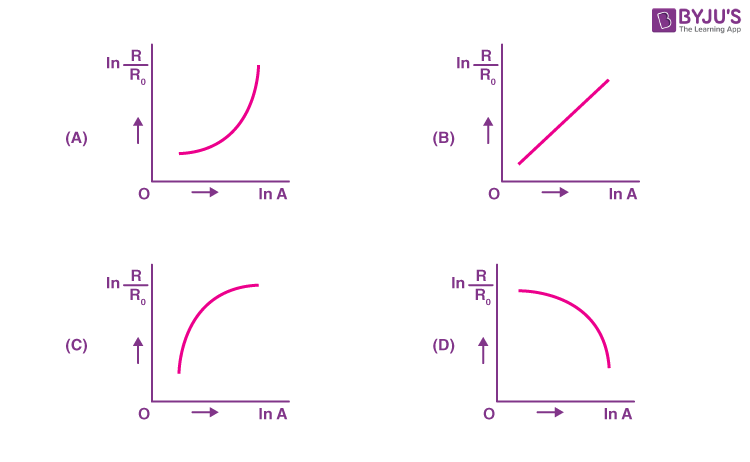Sol. We know that

R = R0A1/3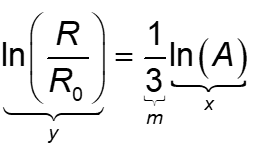⇒ Straight line

18. Identify the logic operation performed by the given circuit: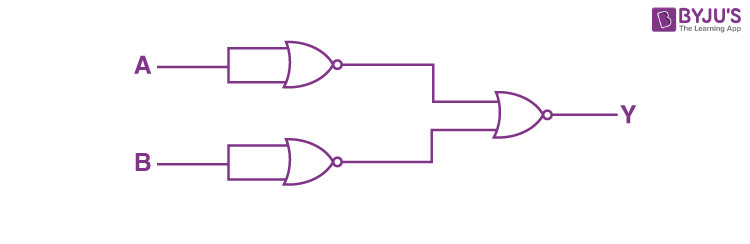(A) AND gate

(B) OR gate

(C) NOR gate

(D) NAND gate

Sol. According to the circuit,

Y = (A′ + B′)′

Y = AB

⇒ AND gate

19. Match List I with List II

 List I List II A. Facsimile I. Static Document Image B. Guided media Channel II. Local Broadcast Radio C. Frequency Modulation III. Rectangular wave D. Digital Single IV. Optical Fiber

Choose the correct answer from the following options:

(A) A-IV, B-III, C-II, D-I

(B) A-I, B-IV, C-II, D-III

(C) A-IV, B-II, C-III, D-I

(D) A-I, B-II, C-III, D-IV

Sol. The correct match is:

 Facsimile – Static Document Image Guided Media Channel – Optical Fiber Frequency Modulation – Local Broadcast Radio Digital single – Rectangular Wave

20. If n represents the actual number of deflections in a converted galvanometer of resistance G and shunt resistance S. Then the total current I when its figure of merit is K will be

$$\begin{array}{l}(A)\ \frac{KS}{S+G}\end{array}$$
$$\begin{array}{l}(B)\ \frac{G+S}{nKS}\end{array}$$
$$\begin{array}{l}(C)\ \frac{nKS}{(G+S)}\end{array}$$
$$\begin{array}{l}(D)\ \frac{nK(G+S)}{S}\end{array}$$

Sol. According to the information, current through galvanometer = nK$$\begin{array}{l}\Rightarrow \frac{S}{S+G}i=nK\end{array}$$
$$\begin{array}{l}\Rightarrow i=\frac{nK(S+G)}{S}\end{array}$$

#### SECTION – B

Numerical Value Type Questions: This section contains 10 questions. In Section B, attempt any five questions out of 10. The answer to each question is a NUMERICAL VALUE. For each question, enter the correct numerical value (in decimal notation, truncated/rounded-off to the second decimal place; e.g. 06.25, 07.00, –00.33, –00.30, 30.27, –27.30) using the mouse and the on-screen virtual numeric keypad in the place designated to enter the answer.

1. For

$$\begin{array}{l}z=a^2x^3y^{\frac{1}{2}},\end{array}$$
where ‘a‘ is a constant. If percentage error in measurement of ‘x‘ and ‘y’ are 4% and 12%, respectively, then the percentage error for ‘z‘ will be _______ %.

Sol. % error in z =

$$\begin{array}{l}3\times 4+\frac{1}{2}\times12\end{array}$$

= 12 + 6 = 18%

2. A curved in a level road has a radius 75 m. The maximum speed of a car turning this curved road can be 30 m/s without skidding. If radius of curved road is changed to 48 m and the coefficient of friction between the tyres and the road remains same, then maximum allowed speed would be ______ m/s.

Sol.

$$\begin{array}{l}\because v=\sqrt{\mu gr} \end{array}$$
$$\begin{array}{l}\Rightarrow \frac{v_1}{v_2}=\sqrt{\frac{r_1}{r_2}}\end{array}$$
$$\begin{array}{l}\Rightarrow \frac{30}{v_2}=\sqrt{\frac{75}{48}}=\sqrt{\frac{25}{16}}=\frac{5}{4}\end{array}$$

V2 = 24 m/s

3. A block of mass 200 g is kept stationary on a smooth inclined plane by applying a minimum horizontal force

$$\begin{array}{l}F=\sqrt{x}~N\end{array}$$
as shown in figure.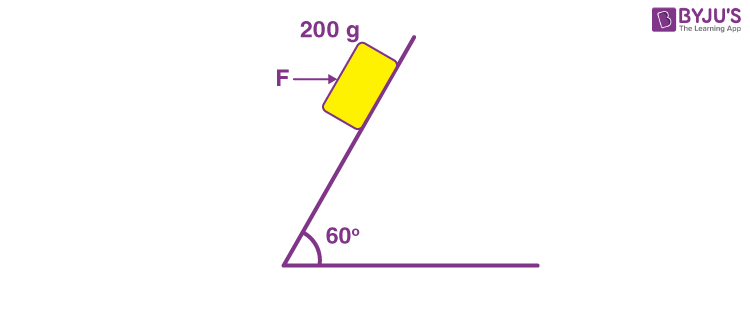The value of x = ______.

Sol.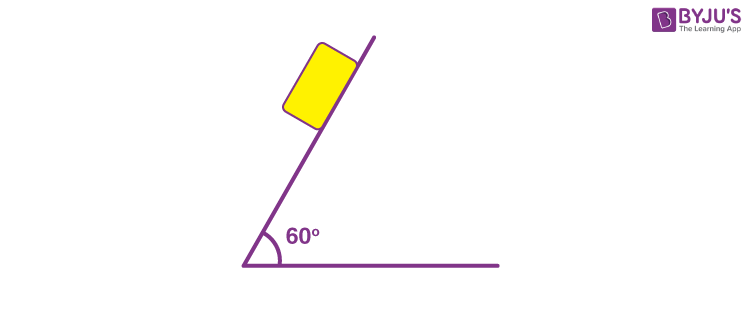F cos60° = mg sin60°

$$\begin{array}{l}F=\frac{1}{2}=0.2\times10\times\frac{\sqrt{3}}{2}\end{array}$$
$$\begin{array}{l}\Rightarrow F=2\sqrt{3}\end{array}$$
$$\begin{array}{l}\Rightarrow F=\sqrt{12}~\text{N}\end{array}$$

x = 12

4. Moment of Inertia (M.I.) of four bodies having same mass ‘M‘ and radius ‘2R‘ are as follows :

I1 = M.I. of solid sphere about its diameter

I2 = M.I. of solid cylinder about its axis

I3 = M.I. of solid circular disc about its diameter.

I4 = M.I. of thin circular ring about its diameter

If 2(I2 + I3) + I4 = x⋅ I1 then the value of x will be _________.

Sol.

$$\begin{array}{l}2\left ( \frac{1}{2}+\frac{1}{4}\right )\times M(2R)^2+\frac{1}{2}M(2R)^2=x\frac{2}{5}M(2R)^2 \end{array}$$
$$\begin{array}{l}\Rightarrow 1+\frac{1}{2}+\frac{1}{2}=x\times\frac{2}{5}\end{array}$$

x = 5

5. Two satellites S1 and S2 are revolving in circular orbits around a planet with radius R1 = 3200 km and R2 = 800 km respectively. The ratio of speed of satellite S1 to the speed of satellite S2 in their respective orbits would be 1/x where x =

Sol.

$$\begin{array}{l}v=\sqrt{\frac{GM}{R}}\end{array}$$
$$\begin{array}{l}\Rightarrow \frac{v_1}{v_2}=\sqrt{\frac{R_2}{R_1}}\end{array}$$
$$\begin{array}{l}\frac{v_2}{v_1}=\sqrt{\frac{3200}{800}}=2\end{array}$$
$$\begin{array}{l}\Rightarrow \frac{v_1}{v_2}=\frac{1}{2}\end{array}$$

x = 2

6. When a gas filled in a closed vessel is heated by raising the temperature by 1ºC, its pressure increases by 0.4%. The initial temperature of the gas is _____ K.

Sol. PV = nRT

So,

$$\begin{array}{l}\frac{dP}{P}\times100=\frac{dT}{T}\times100\end{array}$$
$$\begin{array}{l}0.4=\frac{1}{T}\times100\end{array}$$

T = 250 K

7. 27 identical drops are charged at 22 V each. They combine to form a bigger drop. The potential of the bigger drop will be ______ V.

Sol. Let the charge on one drop is q and its radius is r.

So for one drop

$$\begin{array}{l}V=\frac{Kq}{r}\end{array}$$

For 27 drops merged new charge will be Q = 27q

and new radius is R = 3r

So new potential is

$$\begin{array}{l}V’=\frac{kQ}{R}=9\frac{kq}{r}=9\times22~\text{V}\end{array}$$
= 198 V

8. The length of a given cylindrical wire is increased to double of its original length. The percentage increase in the resistance of the wire will be ______%.

Sol. Volume is constant so on length doubled

Area is halfed so

$$\begin{array}{l}R=\rho \frac{l}{A}\end{array}$$
and
$$\begin{array}{l}R’=\rho \frac{2l}{\frac{A}{2}}=4\rho \frac{l}{A}=4R\end{array}$$

So percentage increase will be

$$\begin{array}{l}R\%=\frac{4R-R}{r}\times100=300\%\end{array}$$

9. In a series LCR circuit, the inductance, capacitance and resistance are L = 100 mH, C = 100 μF and
R = 10 Ω respectively. They are connected to an AC source of voltage 220 V and frequency of 50 Hz. The approximate value of current in the circuit will be ______ A.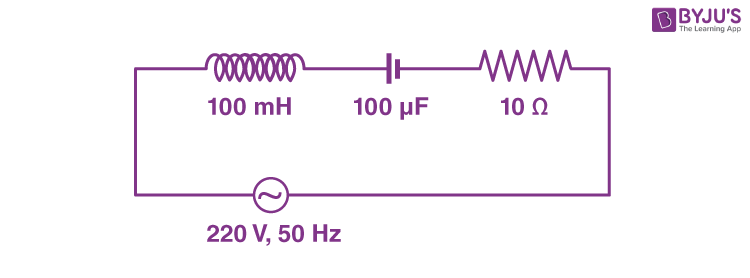Sol.

$$\begin{array}{l}Z=\sqrt{R^2+(X_L-X_C)^2}\end{array}$$
$$\begin{array}{l}=\sqrt{10^2+\left [ 10\pi-\frac{100}{\pi} \right ]^2}\Omega \end{array}$$

≃10 Ω

⇒ Current

$$\begin{array}{l}=\frac{220}{10}~\textup{A}=22~\textup{A}\end{array}$$

10. In an experiment of CE configuration of npn transistor, the transfer characteristics are observed as given in figure.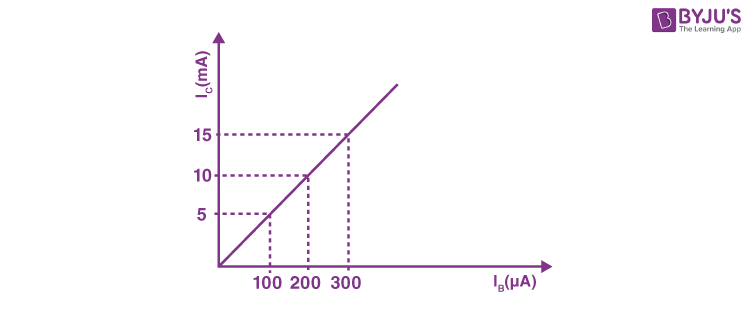If the input resistance is 200 Ω and output resistance is 60 Ω, the voltage gain in this experiment will be_________.

Sol. Voltage gain

$$\begin{array}{l}=\frac{I_CR_0}{I_BR_i}\end{array}$$
$$\begin{array}{l}=\frac{(10~ \textup{mA})(60~\Omega)}{(200 \mu\textup{A})(200~\Omega )}\end{array}$$

⇒ Voltage gain = 15

### Download PDF of JEE Main 2022 June 25 Shift 2 Physics Paper & Solutions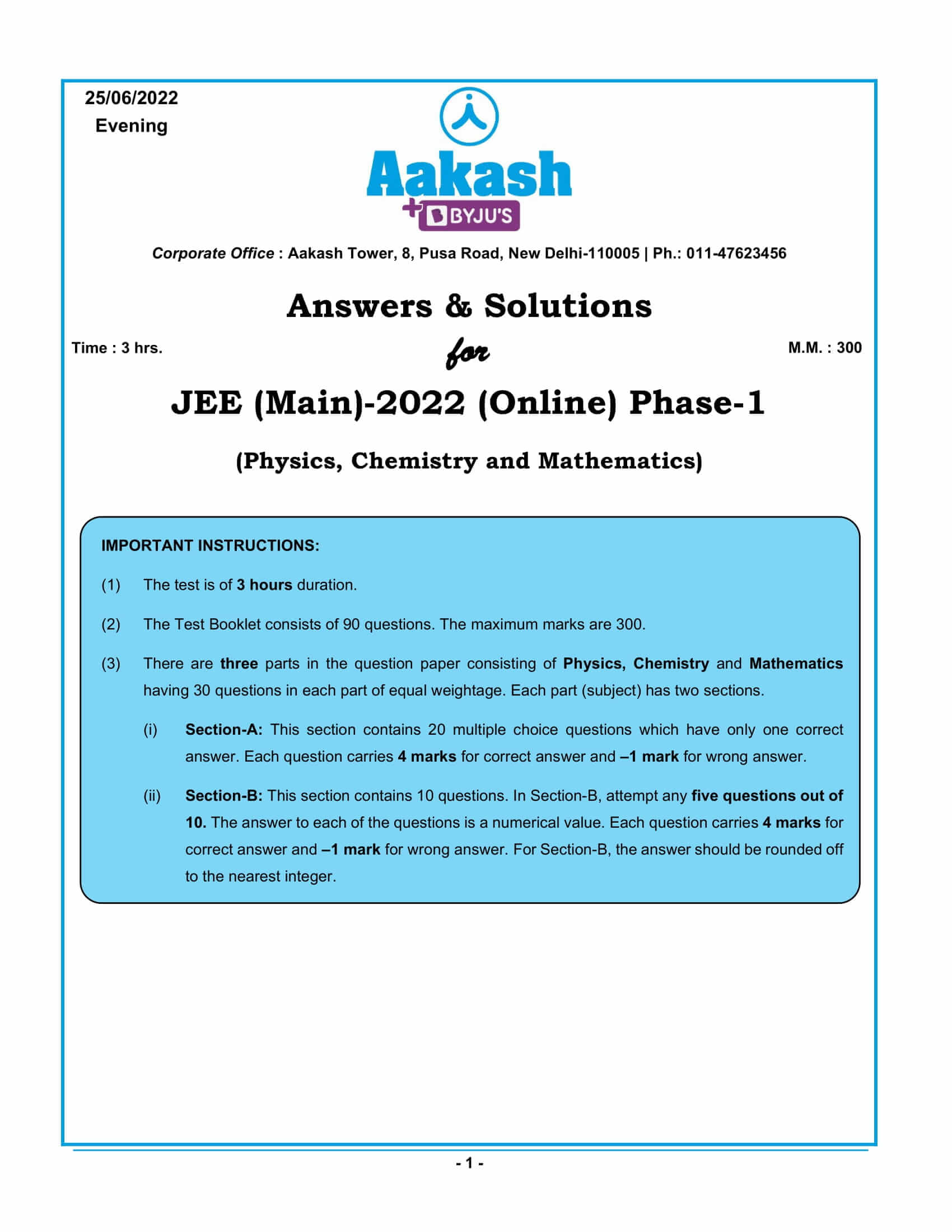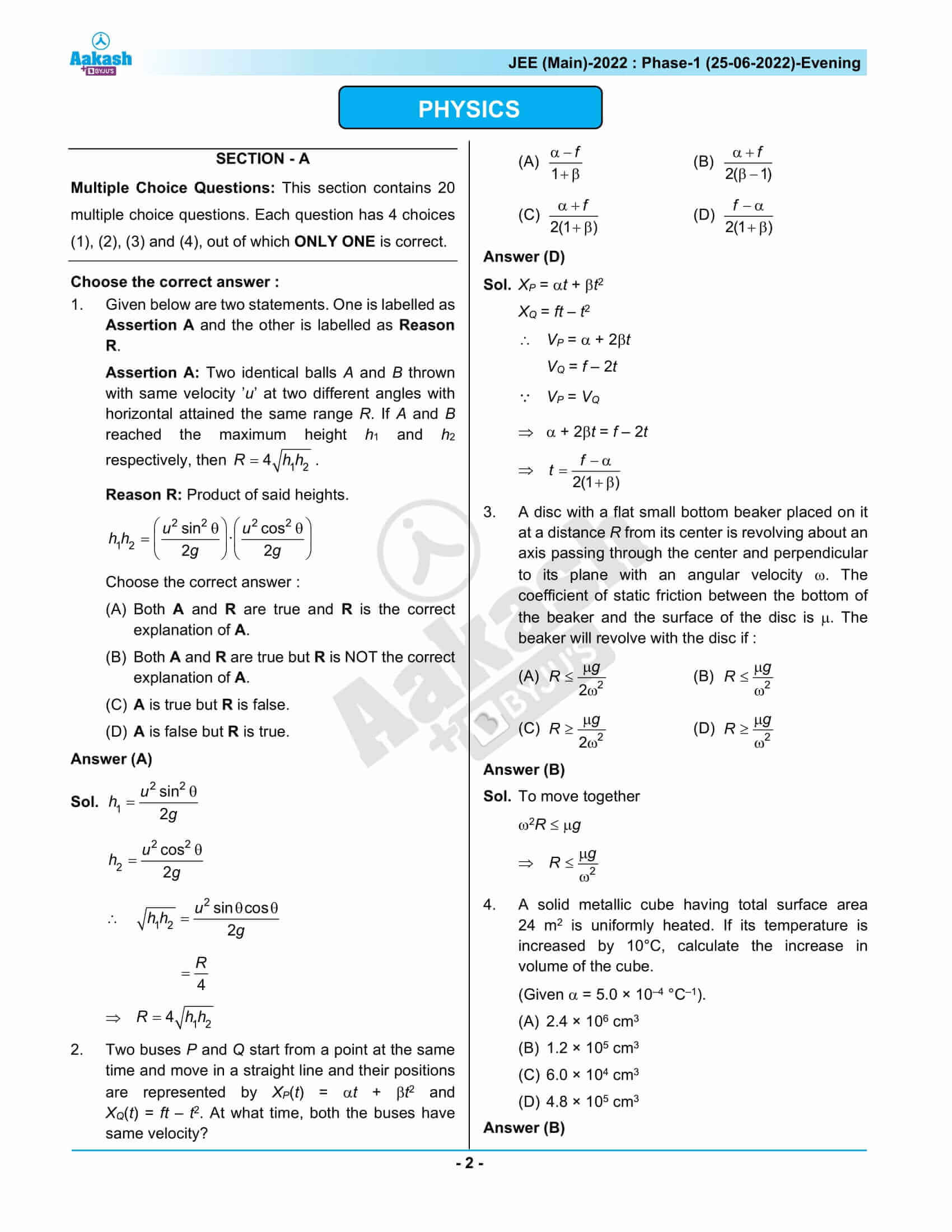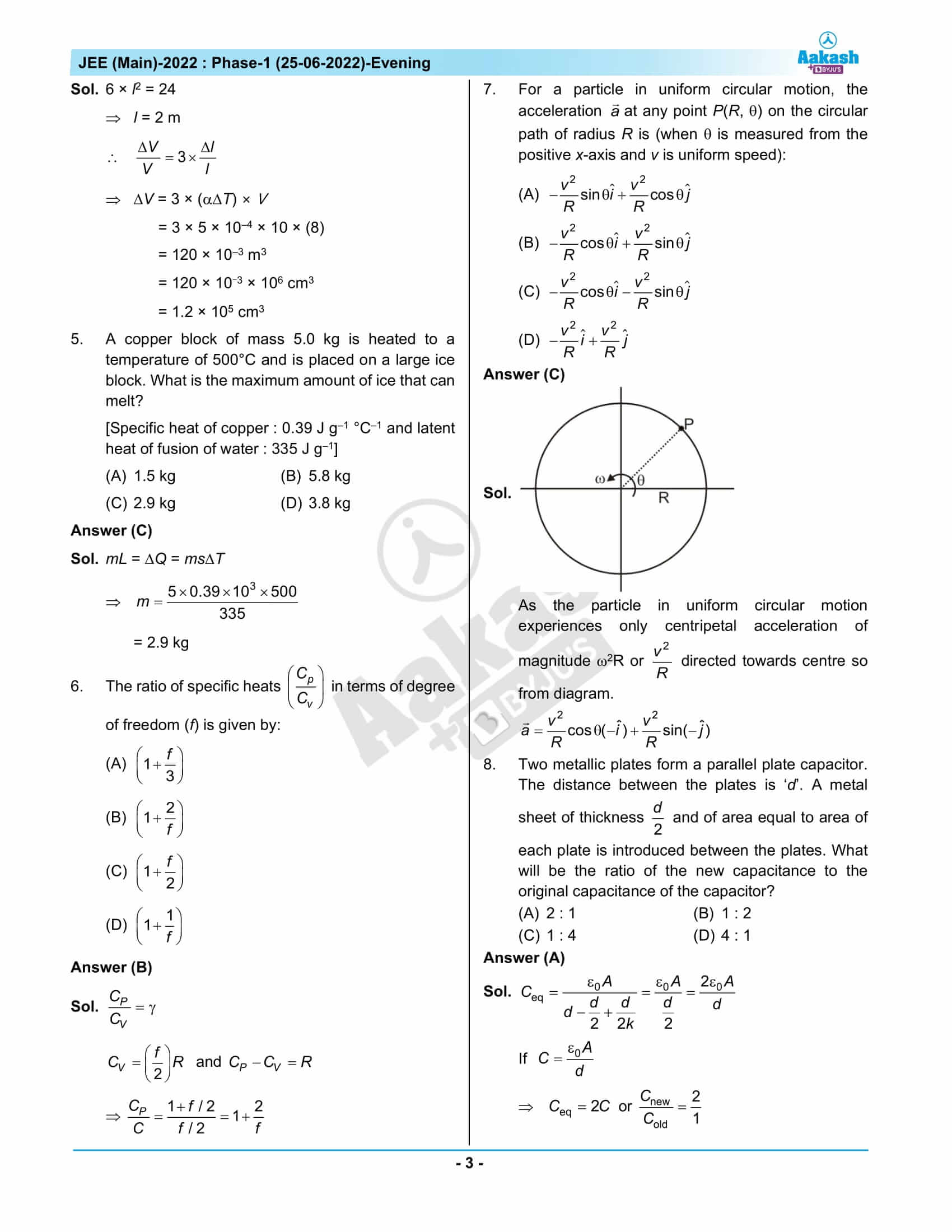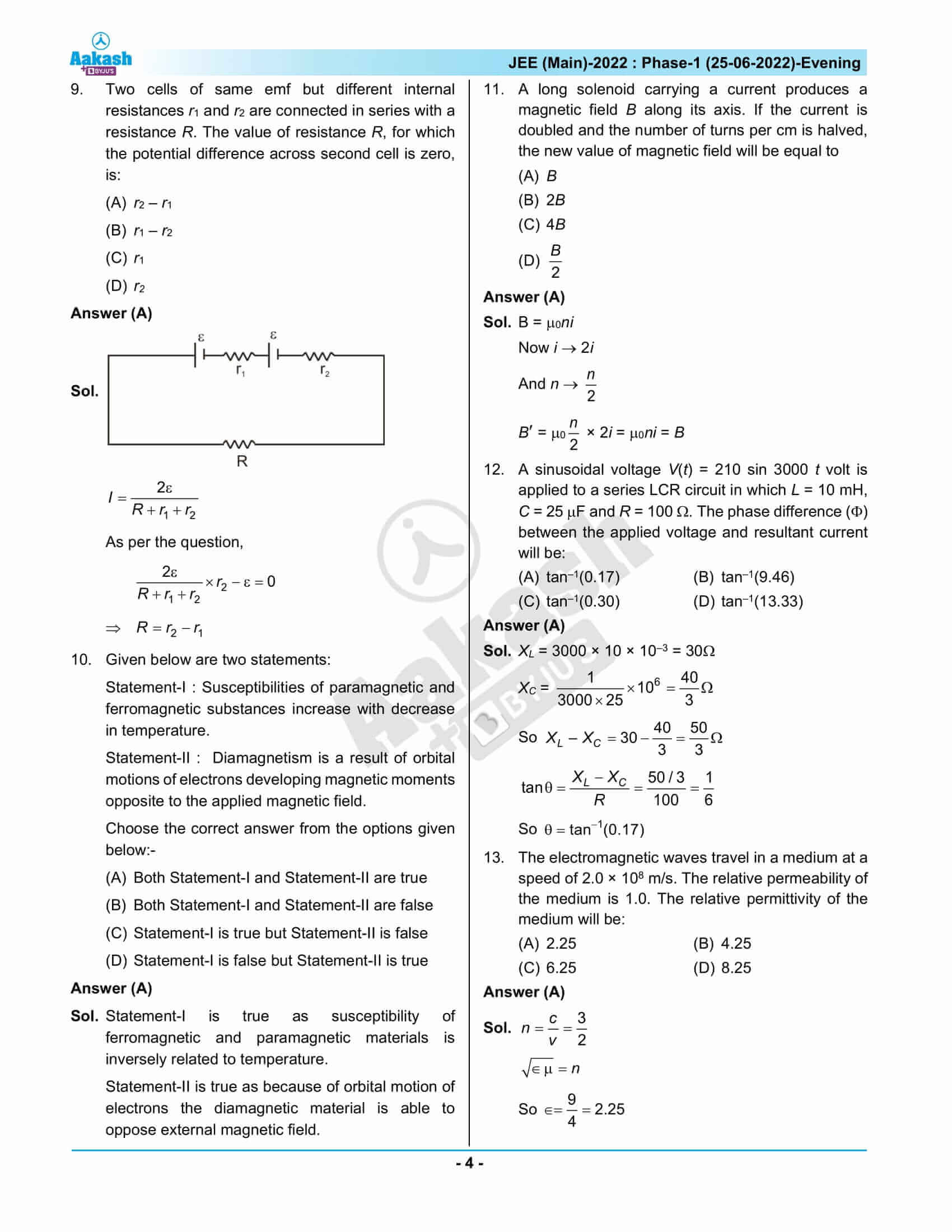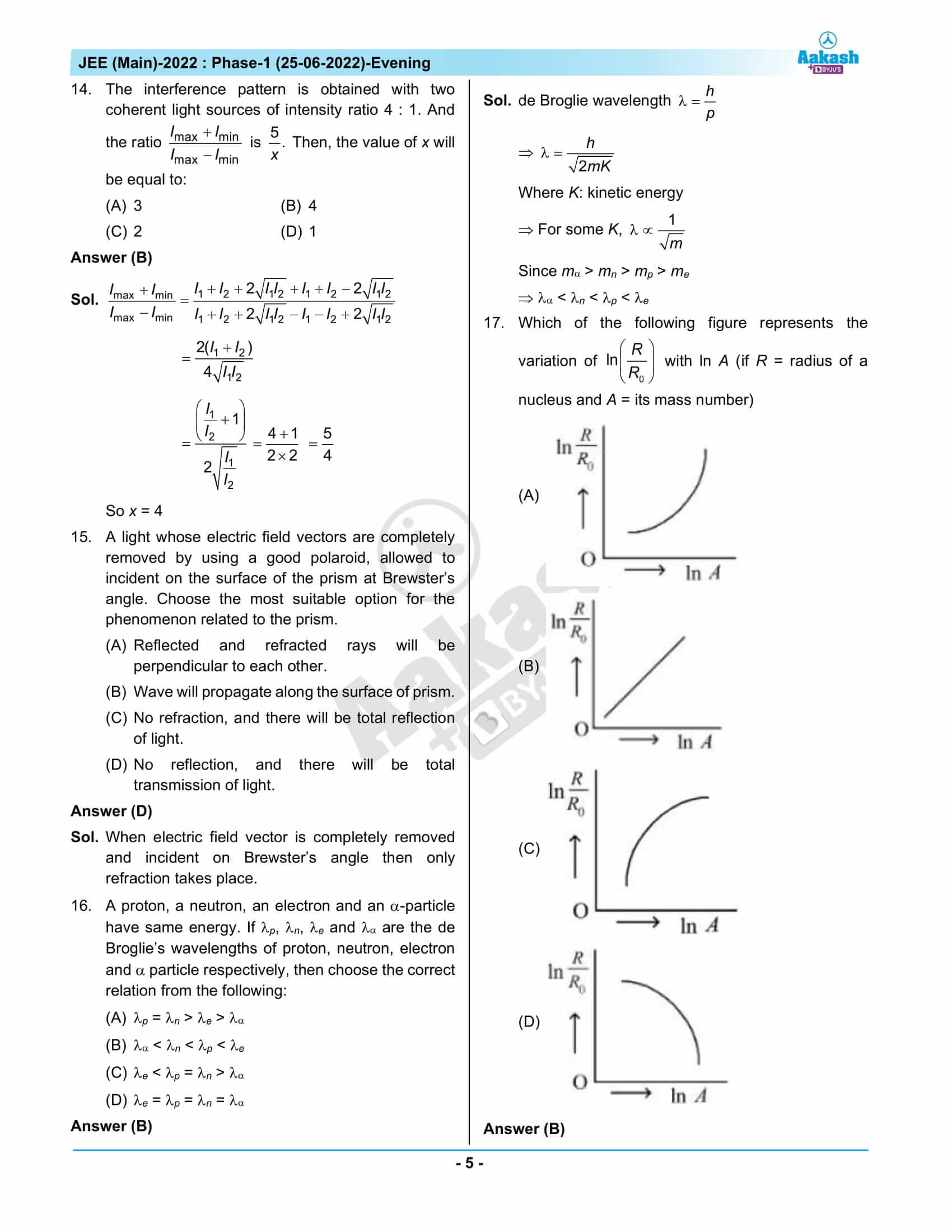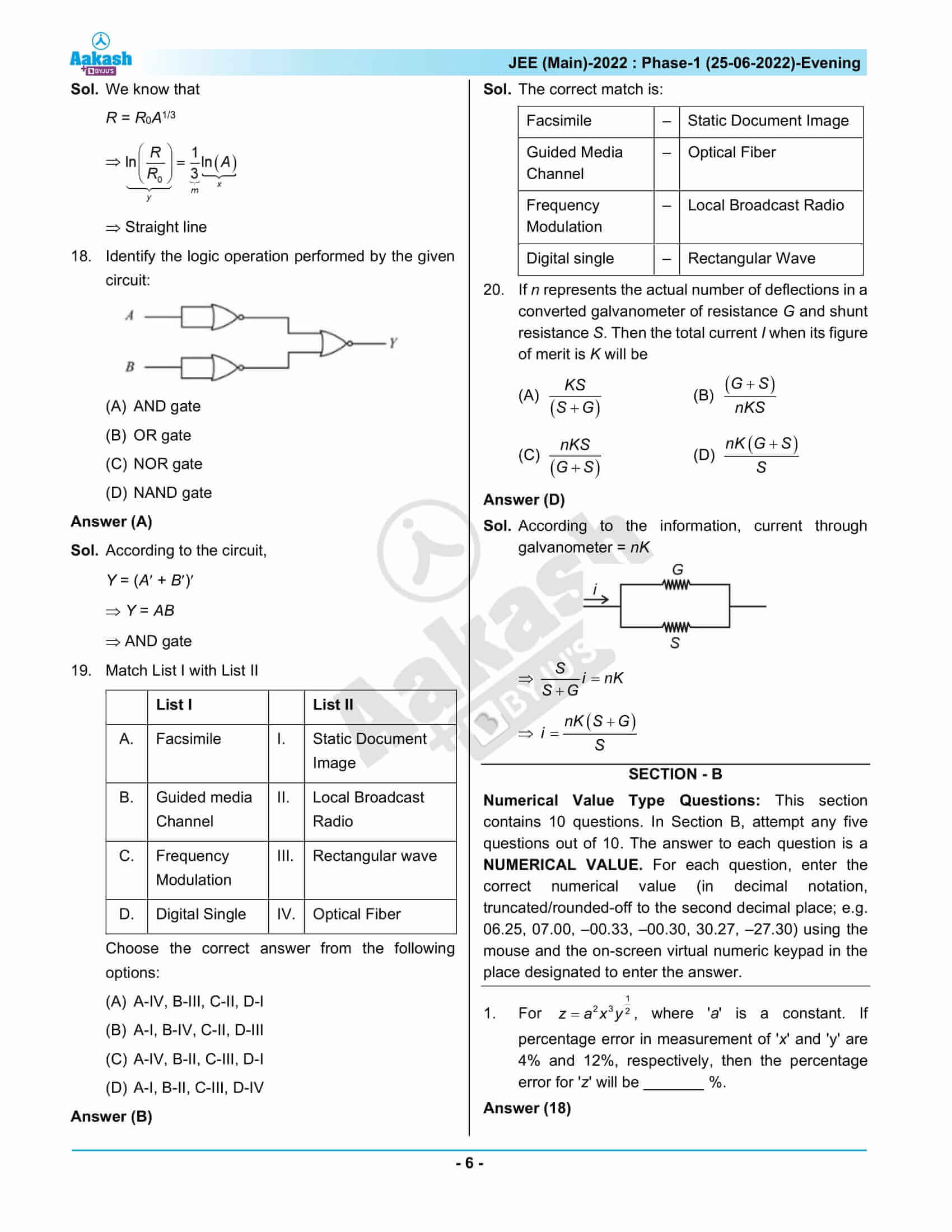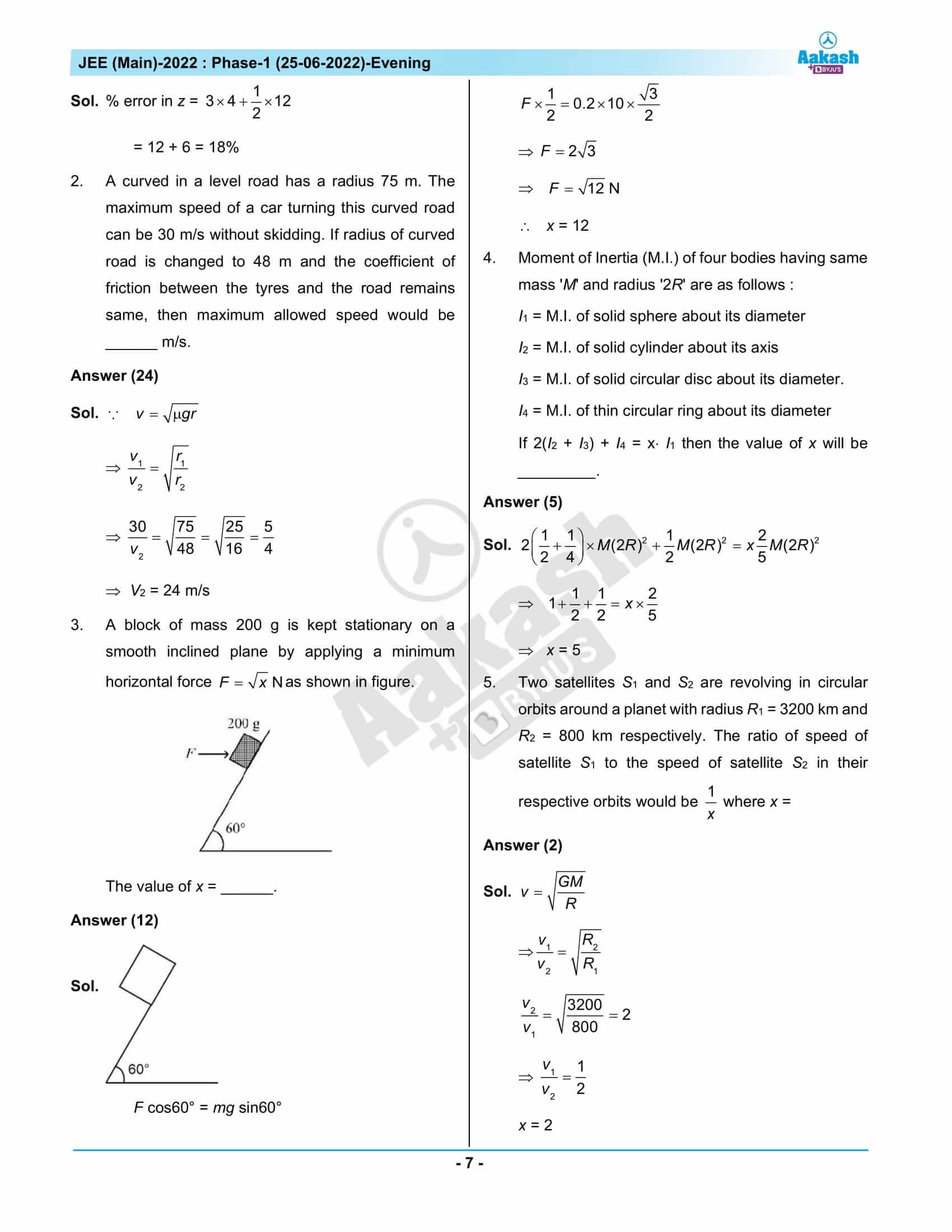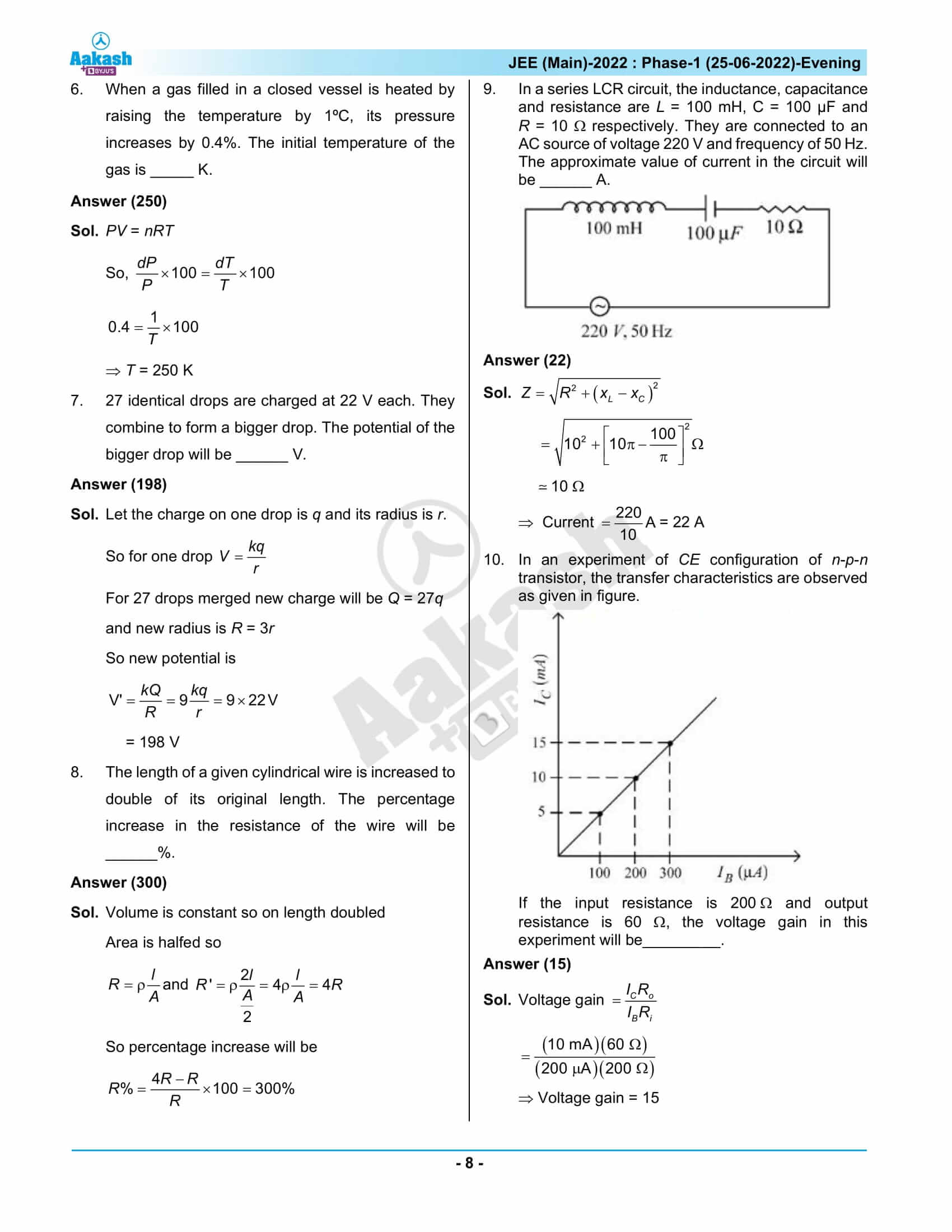## JEE Main 2022 Question Paper & Solutions – 25th June Shift 2

### JEE Main 2022 June 25 Shift 2 Question Paper – Physics Solutions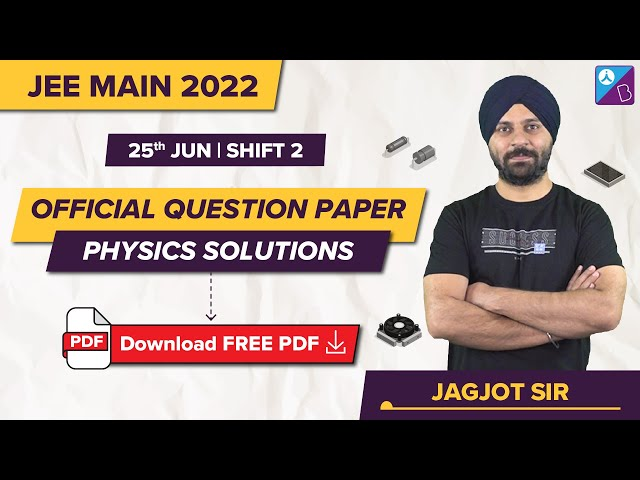### JEE Main 2022 June 25 Shift 2 Question Paper – Chemistry Solutions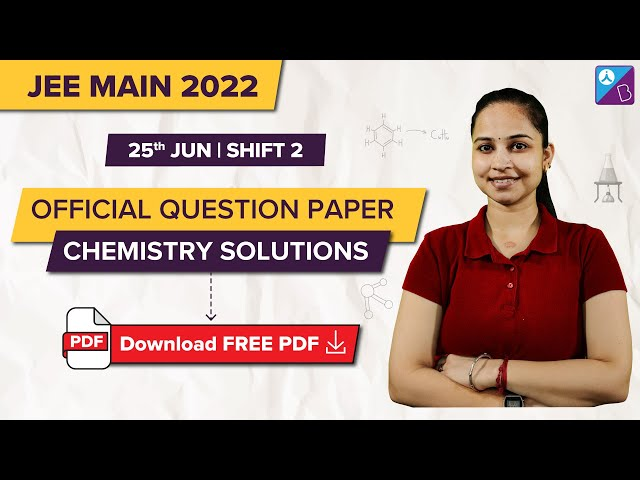### JEE Main 2022 June 25 Shift 2 Question Paper – Maths Solutions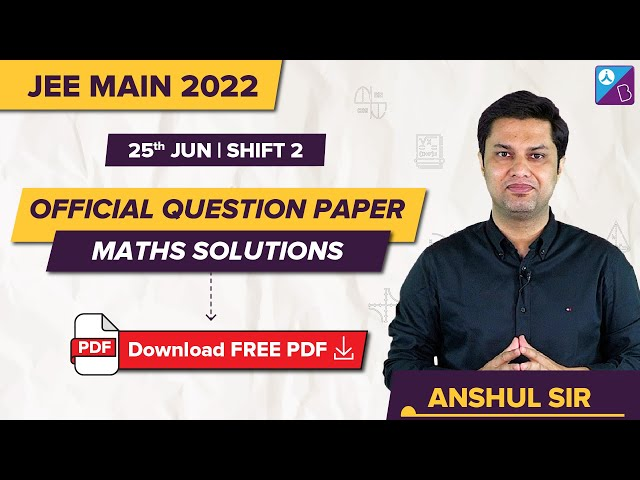## Frequently Asked Questions – FAQs

Q1

### What is the difficulty level of Physics questions in JEE Main 2022 June 25 Shift 2 Question Paper?

Most of the questions from Physics were either easy or of moderate difficulty. The questions asked in Physics are rated 1.5 on a scale of 1 to 3.
Q2

### What is the chapter-wise question distribution for Physics in JEE Main 2022 June 25th shift 2 question paper?

As per our expert faculties, the questions from the Class 12th syllabus and Class 11th syllabus had an approximate equal weightage for all the subjects. Considering only the 22 memory-based Physics questions, 30 % of the questions were from the Class 11 syllabus, and 43 % of the questions were from the Class 12 syllabus.
Q3

### What was the difficulty level of the questions asked from the topic Dual Nature of Radiation and Matter in JEE Main 2022 June 25 – Shift 2 Question Paper?

The questions asked in Dual Nature of Radiation and Matter were easy.
Q4

### How many Physics questions were difficult in JEE Main 2022 25th June second shift question paper?

Physics had 12 easy questions and 9 medium-type questions and 1 difficult question as per the expert analysis on the memory-based question paper.
Q5

### How many Physics questions were asked in the 25th June shift 2 JEE Main question paper 2022?

There were 30 Physics questions asked in the 25th June shift 2 JEE Main question paper 2022.